Start typing, then use the up and down arrows to select an option from the list.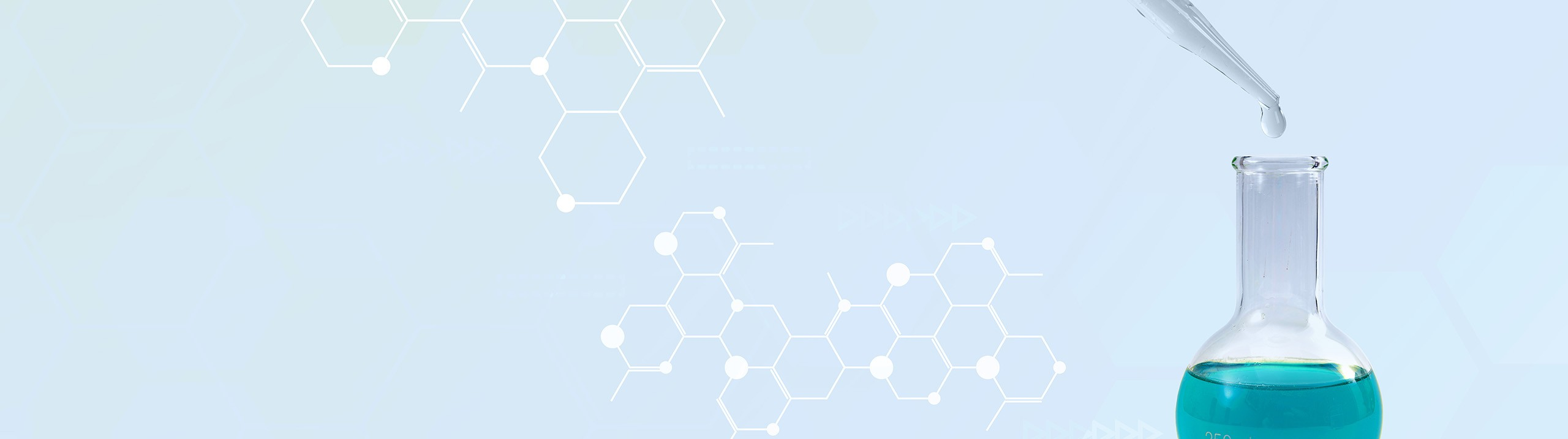# Analytical Chemistry

Learn the toughest concepts covered in your Analytical Chemistry class with step-by-step video tutorials and practice problems.

Chemical Equilibrium

# Le Chatelier's Principle

Le Chatelier's Principle discusses the shifting pattern of a chemical reaction in order to maintain an equilibrium state.

## Chemical Thermodynamics

1

#### concept

Le Chatelier's Principle2m
Play a video: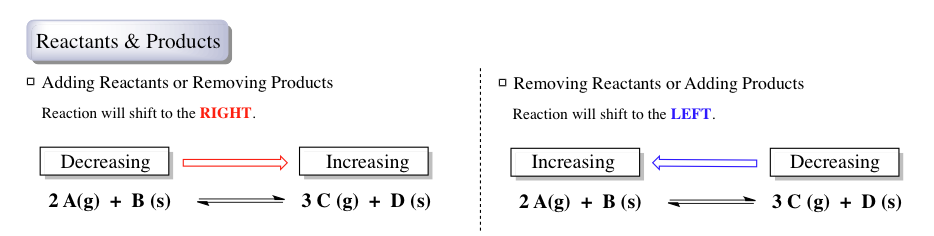2

#### concept

Le Chatelier's Principle2m
Play a video:
Now here we're going to adjust the pressure and volume and see what changes it has on the chemical reaction. So here we're gonna say if we're decreasing pressure, that's the same thing is increasing volume because they're inversely proportional, they're basically opposites of one another. So for decreasing pressure or increasing volume, the re actual shift to the side with more moles of gas. So if we take a look here, we can see that we have two moles of gas, one mole of solid. So overall, here we have two moles of gas, and on this side we have three moles of gas, one mole of solid. So overall we have three moles of gas. So again, if we're decreasing pressure or increasing volume, we're gonna shift to the side with more moles of gas. So in this example we shift in the forward direction, remember wherever we're shifting will be increasing in amount. So we expect our products to be increasing and if the product side is increasing, that means that my reacting side is decreasing. Then if we do the opposite here we're increasing the pressure, which means that we're decreasing the volume. So the reaction would shift to the side with less moles of gas. Again, we still have two moles of gas overall here, three moles of gas here, we're going to shift to the side with less moles of gas. So the side that has two moles of gas in this example, wherever we're shifting will be increasing an amount and if that's increasing amount, that means the other side is decreasing an amount. So remember if we're affecting pressure and volume, we're gonna look and see what sides have either more moles of gas or less moles of gas. If both sides have an equal number of gas molecules, then no shift will occur, because again, we're always looking to see do we shift to the side with more moles or less moles of gas? And if both sides are equal, there's no direction we can shift. Not that we've seen this one will move on to the effects of temperature in terms of shifting a chemical reaction. So click onto the next video and see how temperature plays a role in the chandeliers principle.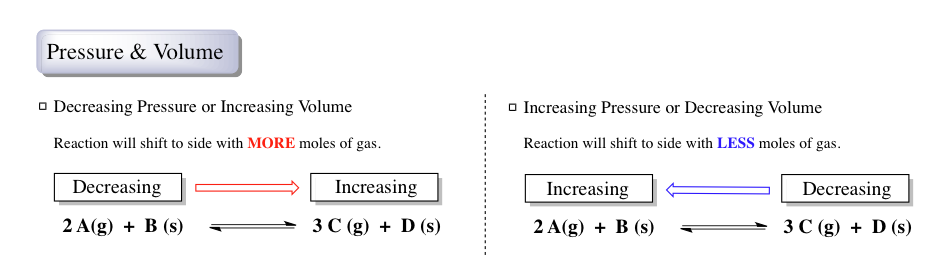3

#### concept

Le Chatelier's Principle2m
Play a video:
now whenever we're dealing with temperature and how it affects the rattlers principle we first have to determine is our reaction, eggs are thermic or endo thermic. So in X. A thermic reaction has a negative delta H. Or envelop here, heat would be a product because in an exotic thermic process we release heat. So heat will be a product if delta H. Is positive. That means we're dealing with an indo thermic process. So that means heat will be reactant in an endo thermic process. We absorb heat. So heat will be a reactant. Now if we're increasing the temperature, if you're increasing the temperature the reaction will shift away from heat. Yeah and again you need to determine if it's an XR thermic or endo thermic process. So you can see what side heat is on. In this example we're looking at an endo thermic process because heat is a reactant, we're increasing temperature. So we're gonna shift away from heat. So we move to the right side because that will be away from the heat source wherever we're shifting to will be increasing amount. And then and then this side would have to be decreasing an amount. So again you can't determine which way reaction will shift until you first figure out is an endo thermic or exhaust thermic. Now here for decreasing the temperature reaction will shift towards heat. So heat here is still on the reacting side. So this is still an endo thermic process. So here we would shift this way towards my heat. So that would mean that this year would be increasing and this side here would have to be decreasing. So whether temperature is increasing or decreasing is not enough information you first have to determine. Is it eggs a thermic or endo thermic? Place the heat on the appropriate side, whether it's reactant for endo thermic or whether it's a product for exa thermic. Once you determine the side the heat is on, then you can look and see okay, what effect will increasing or decreasing the temperature have in terms of shifting this chemical reaction based on the chandeliers principle. So a few factors down. We'll go on to inert gasses in the next video. So click on the next video and see what happens when we're dealing with inert gasses, chandeliers principle.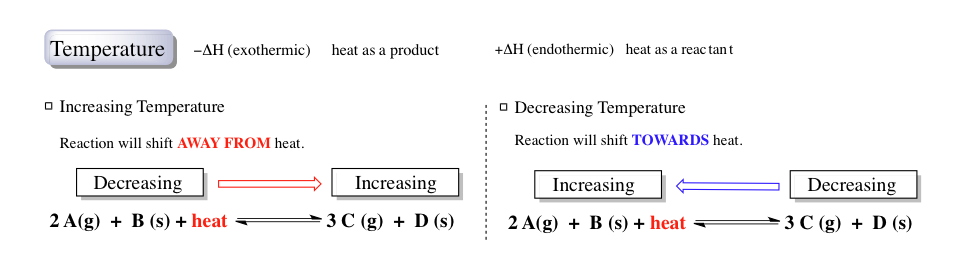4

#### concept

Le Chatelier's Principle2m
Play a video: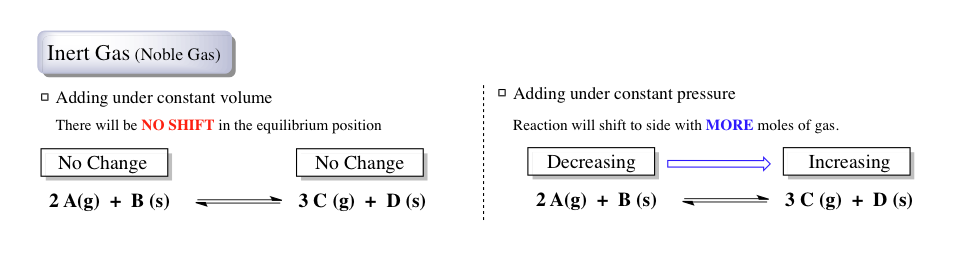Under equilibrium conditions, the addition or removal of a liquid, solid or catalyst will cause no shift in the reaction's position.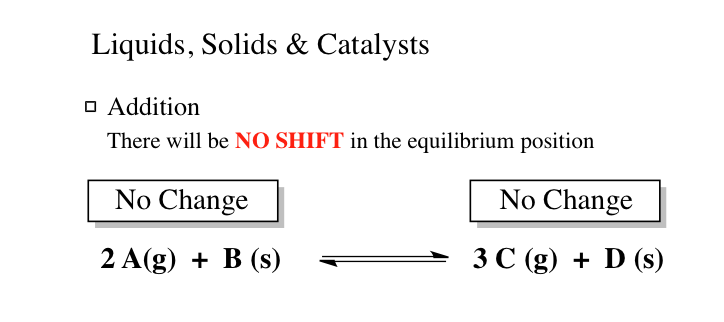5

#### example

Le Chatelier's Principle4m
Play a video:
So here it says the following data was collected for the following reaction at Equilibrium. Hayward told that 55°C, our equilibrium constant K is 4.7 times 10 to the -7. And at 100°C, K now becomes 1.9 times 10 to the negative. To where asked which of the following statements is true. Alright, so remember, the only real factor that can affect my equilibrium constant is temperature. Here we see that our temperature is increasing. So temperature is increasing. Now we have rules in terms of the Chancellor's principle that discuss what happens when we affect temperature. And according to the Chancellor's principle, it says that when we increase temperature, we're gonna shift away from heat. Okay, so that's what the increasing temperature is telling us. Now, we have to figure out what side is heat on. How can we determine which side heat is on? We can determine that by figuring out which way does my chemical reaction shift. If we take a look at 55 degrees Celsius, your equilibrium constant is 4.7 times 10 to the negative seven. When we increase the temperature it actually increases. Now it's 1.9 times 10 to the negative two. So we're gonna say here, if you're increasing your equilibrium constant. K. That gives us a clear indication of what of which direction our reaction is shifting. So think about it. K equals products. Overreacting. The only way for K to increase is from my product amount to go up to increase and my reacting amount to decrease. So when K is increasing, that means products are increasing and react ints. Mhm are decreasing. Okay, so think about it. How can that happen in terms of shifting in a chemical reaction? Well, if my products are increasing, that must mean my reaction is shifting towards them. So we must be heading in the four directions so that my products are increasing and so that this side here is decreasing. So looking at K if it's increasing or decreasing gives me the direction involved. We figured out that we're heading in the forward direction, going back to temperature, we would be shifting away from heat. So for shifting in the four direction, that must mean that heat is a reactant, it's on the reacting side. So we normally shift away from it with the increase in temperature. So if heat is a reactant, that must be my reaction is endo thermic a positive delta H value here. So A. Is out. The entropy change for DELTA H is equal to zero. So if DELTA H is equal to zero, that means that we're thermal neutral. If we're thermal neutral, that would mean that increasing the temperature or decreasing the temperature would cause no change in my equilibrium constant. So K would stay the same exact value. If we were indeed thermal neutral. Since K is changing when I change temperature, we know that DELTA H cannot be equal to zero. And then finally we did have enough information. We figured out that the reaction is endo thermic. So again, the two things that we look at is we look at the change in temperature to determine um what we're following in terms of the Chancellor's principle and then we look at K. Is it increasing or decreasing? This helps us to determine which direction my chemical reaction will shift. Then apply Chancellor's principle with temperature to determine what side the heat is on now that we've seen this. Um We'll go on to example two in terms of the settlers principle. Um Go ahead and try to see if you can get the answer. If you get stuck. Don't worry. Just come back to the next video and see how I approach example too.
6

#### example

Le Chatelier's Principle2m
Play a video: